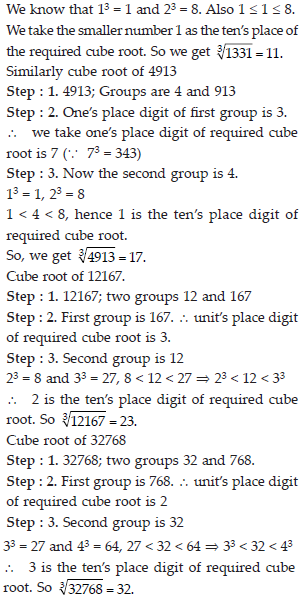# Cubes and Cube Roots (Mathematics) Class 8 - NCERT Questions

Q 1.

Which of the following numbers are not perfect cubes?
(i) 216 (ii) 128 (iii) 1000 (iv) 100 (v) 46656.

SOLUTION: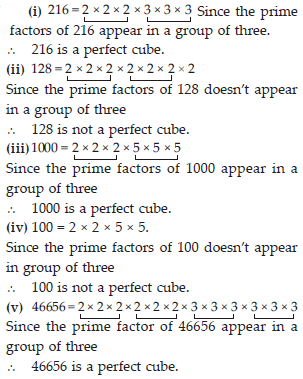Q 2.

Find the smallest number by which each of the following numbers must be multiplied to obtain a perfect cube.
(i) 243 (ii) 256 (iii) 72 (iv) 675 (v) 100.

SOLUTION: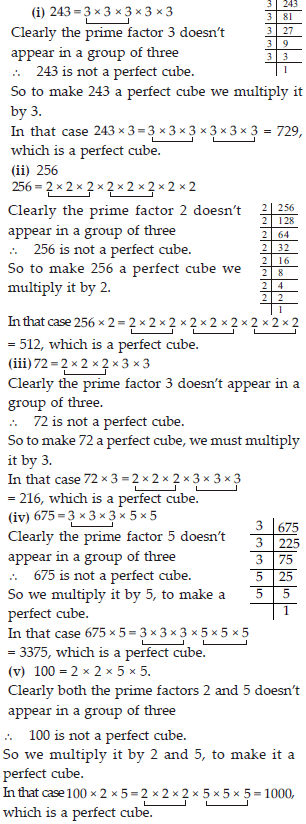Q 3.

Find the smallest number by which each of the following numbers must be divided to obtain a perfect cube.
(i) 81 (ii) 128 (iii) 135 (iv) 192 (v) 704.

SOLUTION: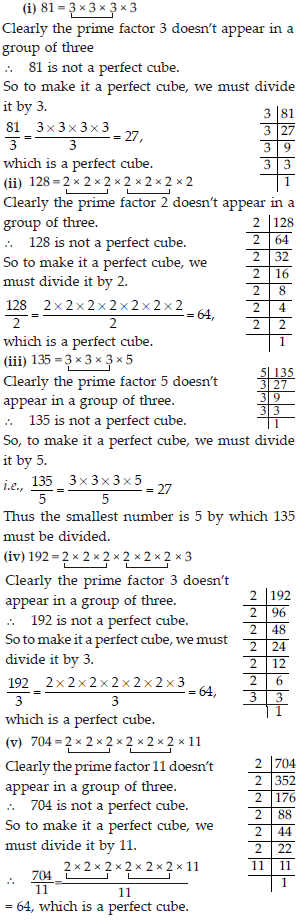Q 4.

Parikshit makes a cuboid of plasticine of sides 5 cm, 2 cm, 5 cm. How many such cuboids will he need to form a cube?

SOLUTION: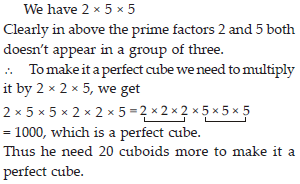Q 5.

Find the cube root of each of the following numbers by prime factorisation method.
(i) 64 (ii) 512 (iii) 10648 (iv) 27000 (v) 15625 (vi) 13824 (vii)110592 (viii) 46656 (ix) 175616 (x) 91125.

SOLUTION: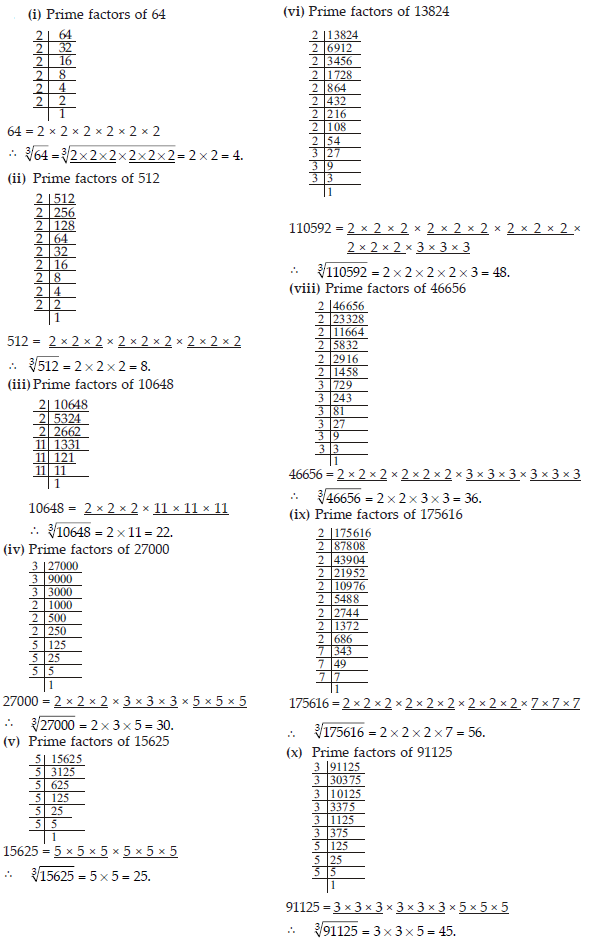Q 6.

State true or false.
(i) Cube of any odd number is even.
(ii) A perfect cube does not end with two zeros.
(iii) If square of a number ends with 5, then its cube ends with 25.
(iv) There is no perfect cube which ends with 8.
(v) The cube of a two digit number may be a three digit number.
(vi) The cube of a two digit number may have seven or more digits.
(vii)The cube of a single digit number may be a single digit number.

SOLUTION: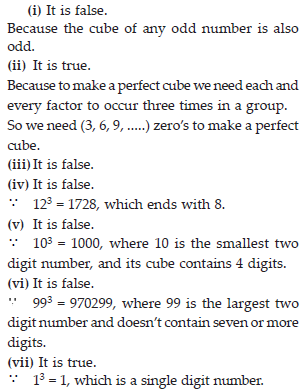Q 7.

You are told that 1,331 is a perfect cube. Can you guess without factorisation what is its cube roots? Similarly, guess the cube roots of 4913, 12167, 32768.

SOLUTION:

The given number is 1331.
Step : 1. Start making groups of digits starting from the right most digit of the number we get 1 and 331 as two groups of one and three digits.
Step : 2. First group i.e., 331 will give the one’s digit (unit’s) of the required cube root.
The number 331 ends with 1. We know that 1 comes at the unit’s place of a number only when its cube root ends in 1.
So, we get 1 at the unit’s place of the cube root. Step : 3. Now take another group i.e., 1